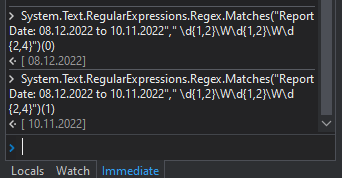# How to get two different dates separately by using Regex

Hi guys,

Here I have to get both the dates using regex, I used \d{1,2}\W\d{1,2}\W\d{2,4} this to get dates but while running the bot it only shows the 1st date as output . how can i get both dates separtely. Can anyone help me?

Use the following

Assign

`Matchcol(Variable type MatchCollection) = System.Text.RegularExpressions.Regex.Matches("YourString","\d{2}\.\d{2}\.\d{4}")`

To access each either use for loop with type argument as System.Text.RegularExpressions.Regex.match

Inside the loop use currentitem.Value to get the values

Or
for first values
`Matchcol(Variable type MatchCollection) = System.Text.RegularExpressions.Regex.Matches("YourString","\d{2}\.\d{2}\.\d{4}")(0).value`
For second value
`Matchcol(Variable type MatchCollection) = System.Text.RegularExpressions.Regex.Matches("YourString","\d{2}\.\d{2}\.\d{4}")(1).value`

cheers

1 Like

You can try with Two Regex expression to get both the date

``````System.Text.RegularExpressions.Regex.Matches(YourString,“\d{2}\d{2}.\d{4}”)(0).Value
``````
``````System.Text.RegularExpressions.Regex.Matches(YourString,“\d{2}\d{2}.\d{4}”)(1).Value
``````1 Like

Hi,

FYI, another approach

If you think readability as important, Named group of regex might be better, as the following.

``````m = System.Text.RegularExpressions.Regex.Match(yourString,"(?<FROM>\d{1,2}\W\d{1,2}\W\d{2,4})\D+(?<TO>\d{1,2}\W\d{1,2}\W\d{2,4})")
``````

Then

``````m.Groups("FROM").Value
m.Groups("TO").Value
``````

Regards,

1 Like

Thanks @Gokul001 It is working

This topic was automatically closed 3 days after the last reply. New replies are no longer allowed.